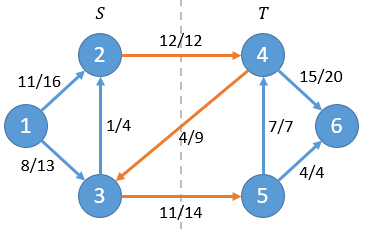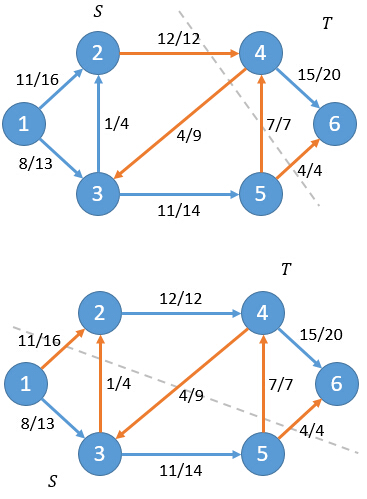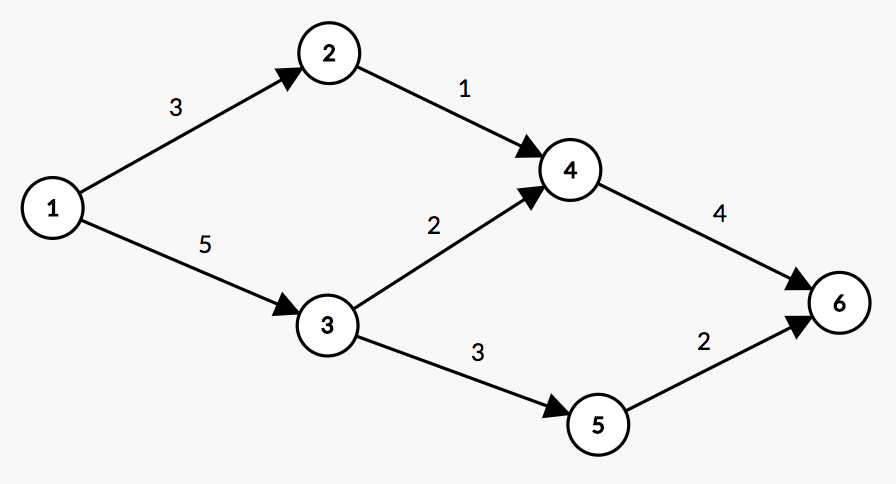# Hihocoder1378 网络流二·最大流最小割定理（最大流+最小割）

## 1378 : 网络流二·最大流最小割定理

### 描述

f(S,T) = Σf(u,v) | u∈S,v∈T净流f = f(2,4)+f(3,4)+f(3,5) = 12+(-4)+11 = 19

C(S,T) = Σc(u,v) | u∈S,v∈T

c(2,4)+c(3,5)=12+14=26f(S,T) = f(S,V) - f(S,S)

f(S,T) = f(S,V)

f(S,T) = f(s,V) + f(S-s,V)

f(S,T) = f(s,V)

f(S,T) = f(s,V) = f

对于一个网络流图G=(V,E)，其中有源点s和汇点t，那么下面三个条件是等价的：
1. 流f是图G的最大流
2. 残留网络Gf不存在增广路
3. 对于G的某一个割(S,T)，此时f = C(S,T)

我们利用反证法，假设流f是图G的最大流，但是残留网络中还存在有增广路p，其流量为fp。则我们有流f'=f+fp>f。这与f是最大流产生矛盾。

假设残留网络Gf不存在增广路，所以在残留网络Gf中不存在路径从s到达t。我们定义S集合为：当前残留网络中s能够到达的点。同时定义T=V-S。

由于f的上界为最小割，当f到达割的容量时，显然就已经到达最大值，因此f为最大流。

### 样例输入

6 7
1 2 3
1 3 5
2 4 1
3 4 2
3 5 3
4 6 4
5 6 2

### 样例输出

5 4
1 2 3 5

### 链接

https://hihocoder.com/problemset/problem/1378

### 题意### 代码

StatusAccepted
Time32ms
Memory4096kB
Length2521
#include <iostream>
#include <cstdio>
#include <cstring>
#include <algorithm>
#include <queue>
#include <vector>
#include <set>
using namespace std;
const int maxn = 510;

int n, m;
struct Edge
{
int u, v, cap;
Edge() {}
Edge(int u, int v, int cap): u(u), v(v), cap(cap) {}
}edge[maxn*maxn];

int R, S, T;
vector <int> E[maxn]; //边集
int dis[maxn];
int current[maxn];
vector <int> V;
bool flag;

void addedge(int u, int v, int cap)
{
E[u].push_back(R);
edge[R++] = Edge(u, v, cap); // 正向边
E[v].push_back(R);
edge[R++] = Edge(v, u, 0); // 方向边容量为0
// 正向边下标通过异或就得到反向边下标, 2 ^ 1 == 3 ; 3 ^ 1 == 2，R从0开始
}

int bfs()
{
memset(dis, 0x3f, sizeof(dis)); // 初始化为0x3f3f3f3f
queue <int> Q;
Q.push(S);
dis[S] = 0;
if (flag)
V.push_back(S);
while (!Q.empty())
{
int u = Q.front();
Q.pop();
for (int i = 0; i < E[u].size(); ++i)
{
Edge &e = edge[E[u][i]];
if (e.cap > 0 && dis[e.v] == 0x3f3f3f3f)
{
dis[e.v] = dis[u] + 1;
Q.push(e.v);
if (flag)
V.push_back(e.v);
}
}
}
return dis[T] < 0x3f3f3f3f; // 返回是否能够到达汇点
}

int dinic(int u, int maxflow)
{
if (u == T)
return maxflow;
for (int i = current[u]; i < E[u].size(); ++i) // 当前弧优化，保存u点增广到了第几条边
{
current[u] = i;
Edge &e = edge[E[u][i]];
if (dis[e.v] == dis[u] + 1 && e.cap > 0)
{
int flow = dinic(e.v, min(maxflow, e.cap));
if (flow)
{
e.cap -= flow; // 正向边流量减少
edge[E[u][i] ^ 1].cap += flow; // 方向边流量增加
return flow;
}
}
}
return 0;
}

int DINIC()
{
int ans = 0;
while (bfs())
{
int flow;
memset(current, 0, sizeof(current)); // bfs后清空当前弧数组
while (flow = dinic(S, 0x3f3f3f3f)) // 一次bfs可以进行多次增广
ans += flow;
}
return ans;
}

int main()
{
int t, u, v, cap;
scanf("%d%d", &n, &m);
R = 0, S = 1, T = n; // 边序号，源点，汇点
for (int i = 0; i <= n; ++i)
E[i].clear();
V.clear();
memset(edge, 0, sizeof(edge));
for (int i = 0; i < m; ++i)
{
scanf("%d%d%d", &u, &v, &cap);
}
int ans = DINIC();
flag = true; // 跑完最大流后再bfs一遍得到S点集
bfs();
printf("%d %d\n", ans, V.size());
printf("%d", V);
for (int i = 1; i < V.size(); ++i)
printf(" %d", V[i]);
return 0;
}

Dinic的效率还是比较高的。

The end.
2018-07-22 星期日